Next: Other Similarity Matrices Up: Point Accepted Mutations and Previous: Percent Identity and PAM

# Estimating Mutation Matrices

This section describes a method for computing mutation matrices, and hence Dayhoff matrices, which is more accurate and based on better theoretical grounds. This method will derive the information from a sample of matches of sequences (see Analysis of Mutation During Divergent Evolution'' M. Cohen, S. Benner and G. Gonnet, ). We will assume that we have a large enough sample which has been inspected and approved by an expert in the area. Although we could construct sample sets automatically, it is important that a person analyses this sample and weeds out unsuitable sequences which do not represent mutations by evolution.

We will further require that this sample be selected so that all its matches have approximately the same PAM distance. For example, let us suppose that all the matches have been selected so that their distance is between 35 and 45 PAM units. Chapter- The Pairwise Comparison of Amino Acid Sequences explains how to compute PAM distances of matches.

Suppose that we run the following experiment:

i)
Generate a random sequence S0.
ii)
Randomly mutate S0 with a 40-PAM mutation matrix generating a new sequence S1.
iii)
Compare S0 and S1 and build a comparison matrix C, such that every time that we have an amino acid i in S0mutating into an amino acid j in S1 we add 1 to Cij(and if it does not mutate, i.e. stays the same, we add 1 to Cii).

It is easy to see that the expected value of C is given by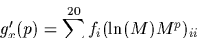where N is a diagonal matrix and Nii is the number of times amino acid i appears in S0. This approximation, by the law of large numbers, will be more accurate as we increase the number of sample points ( we increase the length of S0 and hence increase N).

The above equation is crucial for the estimation of M when we can tabulate a matrix C. Doing some trivial algebra, we find that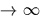Since we do not know from our data which sequence evolved we will add 1/2 to Cij and 1/2 to Cji instead of adding 1 to Cij

The matrix N is obtained from counting all the amino acids analysed. Multiplying C by N-1 is equivalent to dividing each column of C by the sum of the column. (Note the columns of a mutation matrix add up to 1.)

Now, if we were certain about the PAM distance of the sample, in this case 40, we could compute. However, we may not have a lot of trust in the estimated PAM distances. This does not pose a problem since we can use the definition of a 1-PAM matrix as an anchor'':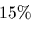The problem reduces to finding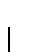such thatis a 1-PAM matrix. This valuewill be an estimate of the average PAM distance of the sample.

The following Darwin function computes an estimate of a 1-PAM matrix based on a sample of matches stored in a file. This function also receives as parameters a minimum value for the similarity, a minimum value for the length of the match and the minimum and maximum value for the PAM distance that we want to select.

> EstMutMat := proc( filename:string, MinSim:real, MinLength:real,
>         Min{PAM}:real, Max{PAM}:real )
> description 'estimate a mutation matrix from matches read from a file';
> C := CreateArray(1..20,1..20);
> M := CreateArray(1..20,1..20);

Print some appropriate message to describe the present run.
> printf('Sample matches from file %s, MinSim=%g, MinLength=%g\n',
>         filename,MinSim,MinLength);
> printf('{PAM} bounded between %3g and %3g\n', MinPAM, MaxPam );

To read a file from Darwin, line by line we first must establish a pipe via the OpenPipe command. This changes the standard input from the keyboard to the specified file. The file can be read line by line by issuing ReadLine calls within the body of a loop.

> OpenPipe('cat filename');

Initialize various counters to zero.
> totm := totma := totlm := toteq := totmut := 0;

This is now the main reading loop. The loop will be exited when the ReadLine call finds an end-of-file in which case returns the string EOF.
> do  m := ReadLine();
>     if m=EOF then break fi;

It is worthwhile to check that we read a match from the file.
>     if not type(m,Match) then error('invalid input in', filename) fi;
>     totm := totm+1;

If the values of the match do not fall within the acceptable ranges, then skip this match.
>     if m[Sim] < MinSim or max(m[Length1],m[Length2]) < MinLength or
>         m[PamNumber] > MaxPam or m[PamNumber] < MinPam then next fi;
>     totma := totma+1;

The function DynProgStrings prepares an alignment for printing. In this case, we are interested in the strings of the aligned sequences as they would be printed. By scanning these two strings we can count the identities and the mutations.
>     sm := DynProgStrings( m, SearchDayMatrix(m[PamNumber],DMS) );
>     lm := length(sm);
>     totlm := totlm + lm;

For each character in the matched strings add in the C matrix.
>     for i to lm do
>         i1 := AToInt(sm[2,i]);  i2 := AToInt(sm[3,i]);
>         if i1>0 and i1<21 and i2>0 and i2<21 then
>             if i1=i2 then toteq := toteq+1;
>                           C[i1,i1] := C[i1,i1]+1
>             else totmut := totmut+1;
>                  C[i1,i2] := C[i1,i2]+1/2;
>                  C[i2,i1] := C[i2,i1]+1/2
>                  fi
>             fi
>         od
>     od;

Print the collected counts.
> printf('%d matches read, %d selected with a total of %d positions,\n',
>         totm, totma, totlm );
> printf('%d exact matches, %d mutations and %d deletions\n\n',
>         toteq, totmut, totlm-toteq-totmut );

Compute the sum of the columns, but since the matrix C is symmetric, it is easier to compute the sum of the rows.
> Cols := CreateArray(1..20);
> for i to 20 do Cols[i] := sum(C[i]) od;

Now compute the frequencies of amino acids into F and normalize C into M.
> tot := sum(Cols);
> F := Cols/tot;
> for i to 20 do for j to 20 do M[i,j] := C[i,j]/Cols[j] od od:

To compute the 1-PAM matrix we need to find the exponent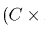that will make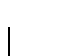a 1-PAM matrix. The following iteration converges quite rapidly to the desired solution. It is based on estimatingas if it were linear on the probability of no change. This is not true, but both values are highly correlated. Hence by estimating and further correcting we converge to the right exponent.
> lnM := ln(M):
> alpha := 1;

Now iterate until a break is executed.
> do  nochange := 0;

Compute the probability of no change and if it is sufficiently close to 1%, exit the loop.
>     for i to 20 do nochange := nochange + F[i]*(1-M[i,i]) od;
>     if abs(nochange-0.01) < DBL_EPSILON then break fi;

If it is not accurate enough, it must be corrected.
>     corr := 0.01/nochange;
>     alpha := alpha/corr;
>     lnM := lnM*corr;
>     M := exp(lnM);
>     od;
> printf('The PAM of the aggregated sample was %5g\n\n',alpha);

Return the logarithm of a 1-PAM matrix and the frequency vector as a list with two elements in it.
> [lnM,F]
> end:


Now run this function with the file SamplePAM40.

> res := EstMutMat( SamplePAM40, 80, 100, 35, 45 ):

Sample matches from file SamplePAM40, MinSimil=80, MinLength=100
PAM bounded between  35 and  45
838 matches read, 838 selected with a total of 244069 positions,
170528 exact matches, 65473 mutations and 8068 deletions

The PAM of the aggregated sample was 33.8193


The PAM estimate of the sample is slightly lower than expected. This indicates that the original Dayhoff matrix tends to overestimate PAM distances.

Now we will compute a new Dayhoff matrix, with PAM 250, so that we may compare it with the original one. Since we have the logarithm of a 1-PAM mutation matrix in res, we can used the CreateDayMatrix command:

> NewD := CreateDayMatrix(res,250):
> print( NewD );
DayMatrix(Peptide, pam=250, Simil: max=15.035, min=-6.237,
max offdiag=5.554, del=-19.814-1.396*(k-1))
C  12.6
S   0.3  2.0
T  -1.1  1.3  2.4
P  -3.1  0.9  0.1  6.8
A  -0.7  1.2  1.1  0.8  2.4
G  -2.1  0.6 -0.6 -1.4  0.8  5.9
N  -1.7  1.0  0.5 -1.1  0.0  0.4  3.2
D  -3.6  0.1 -0.4 -1.6 -0.1  0.6  2.2  4.8
E  -4.2 -0.3 -0.5 -1.1 -0.1  0.1  1.0  3.4  4.2
Q  -3.1 -0.4 -0.4 -0.1 -0.7 -1.2  0.5  0.6  1.7  3.9
H  -1.3 -0.4 -0.9 -0.7 -1.3 -1.7  1.4  0.1 -0.2  2.2  6.1
R  -2.1 -0.4 -0.5 -0.9 -1.2 -0.9  0.2 -1.1 -0.2  2.0  1.5  5.1
K  -3.5 -0.3 -0.1 -1.1 -0.8 -1.1  0.9  0.1  0.9  2.0  0.7  3.5  4.2
M  -1.9 -1.5 -0.1 -2.0 -0.9 -3.4 -2.3 -3.2 -2.6 -1.5 -2.3 -2.0 -1.7  4.8
I  -1.8 -1.4  0.1 -2.1 -0.5 -3.6 -2.4 -3.5 -3.0 -2.5 -2.7 -2.9 -2.7  3.0
L  -2.3 -2.0 -1.0 -1.6 -1.6 -4.4 -3.1 -4.3 -3.6 -1.9 -1.9 -2.5 -2.8  3.2
V  -1.1 -1.0  0.3 -1.5  0.2 -2.4 -2.1 -2.7 -2.2 -2.1 -2.5 -2.7 -2.4  2.3
F  -0.8 -2.1 -2.3 -3.3 -2.9 -5.1 -3.0 -4.8 -4.9 -3.4  0.0 -3.9 -4.5  0.7
Y   0.4 -1.7 -2.4 -3.9 -3.1 -4.3 -1.0 -2.8 -3.6 -2.1  2.8 -2.6 -3.2 -1.7
W  -1.2 -3.5 -4.6 -5.2 -4.6 -3.1 -4.1 -6.2 -5.9 -3.6 -1.7 -0.7 -3.8 -1.8

Although similar, the new matrix has some significant differences with the original one.Next: Other Similarity Matrices Up: Point Accepted Mutations and Previous: Percent Identity and PAM
Gaston Gonnet
1998-09-15